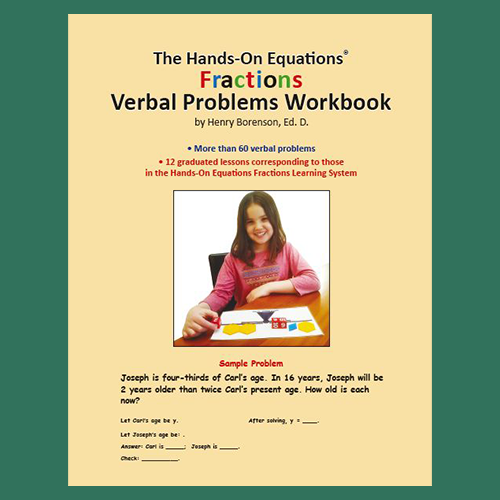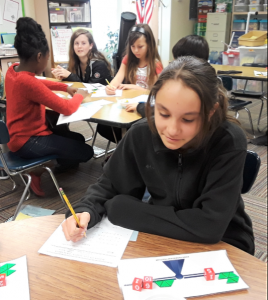# The Hands-On Equations Fractions Verbal Problems Book

\$20.00

SKU: HOEF-VP

## The Hands-On Equations Fractions Verbal Problems Workbook

Each exercise in the twelve lessons of The Hands-On Equations Fractions Verbal Problems Workbook is solved using the strategies presented in the corresponding lesson of The Hands-On Equations Fractions Learning System.

Students concretely represent the verbal problem statement and then simplify the physical setup to solve for the unknown. Worksheet reproduction rights are included.

This workbook is intended for students in grades 5 – 8 and younger gifted students.## Materials needed for each student

Each student needs the HOE Fractions Student Kit to represent and solve the equations concretely. This kit consists of a set of 8 numbered cubes, a flat laminated scale, and a set of 33 plastic fraction blocks.